Nuts

How many we must have at least nuts if we can equally divide it to 10 children, 11 children or 19 children and any nut left?

Result

n =  2090

Solution:Leave us a comment of example and its solution (i.e. if it is still somewhat unclear...):Be the first to comment!To solve this example are needed these knowledge from mathematics:

Do you want to calculate least common multiple two or more numbers?

Next similar examples:

1. Plum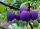On the platter are plum. How many were there if its have to be able to share equally among 8,10 and 12 children?
2. Apples 2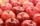How many minimum apples are in the cart, if possible is completely divided into packages of 6, 14 and 21 apples?
3. Seamstress 2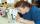Seamstress bought two kinds of textile in whole meters. One at 50 SKK and the second 70 SKK per meter. How many meter bought from this two textiles when paid totally 1540 SKK?
4. 9.A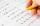9.A to attend more than 20 students but fewer than 40 students. A third of the pupils wrote a math test to mark 1, the sixth to mark 2, the ninth to mark 3. No one gets mark 4. How many students of class 9.A wrote a test to mark 5?
5. CombinationsHow many different combinations of two-digit number divisible by 4 arises from the digits 3, 5 and 7?
6. Divisible by 5How many three-digit odd numbers divisible by 5, which are in place ten's number 3?
7. Two months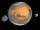Mars has two months, Phobos and Deimos. Phobos orbits around Mars in 7 hours 39 minutes and Deimos in 30 h 14 min. How long will repeat the relative position of the three celestial bodies?
8. Amazing numberAn amazing number is name for such even number, the decomposition product of prime numbers has exactly three not necessarily different factors and the sum of all its divisors is equal to twice that number. Find all amazing numbers.
9. Linear systemSolve a set of two equations of two unknowns: 1.5x+1.2y=0.6 0.8x-0.2y=2
10. Numbers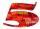Write smallest three-digit number, which in division 5 and 7 gives the rest 2.
11. Theorem proveWe want to prove the sentence: If the natural number n is divisible by six, then n is divisible by three. From what assumption we started?
12. DivisorsThe sum of all divisors unknown odd number is 2112. Determine sum of all divisors of number which is twice of unknown numbers.
13. Unknown numberUnknown number is divisible by exactly three different primes. When we compare these primes in ascending order, the following applies: • Difference first and second prime number is half the difference between the third and second prime numbers. • The produ
14. First manWhat is the likelihood of a random event where are five men and seven women first will leave the man?
15. One halfOne half of ? is: ?
16. Functions f,gFind g(1) if g(x) = 3x - x2 Find f(5) if f(x) = x + 1/2
17. PowersExpress the expression ? as the n-th power of the base 10.